Visualize Word Embeddings with Tensorflow

Word Embeddings are a way to convert words into a numerical representation that machine learning models can use as inputs. There are various methods to generating word embeddings such as bag of words, GloVe, FastText and Word2Vec. Once you have the word embeddings though, how can you visualize them in order to explore the resulting work? In this article you will learn how to visualize word embeddings using the Tensorboard Embedding Projector.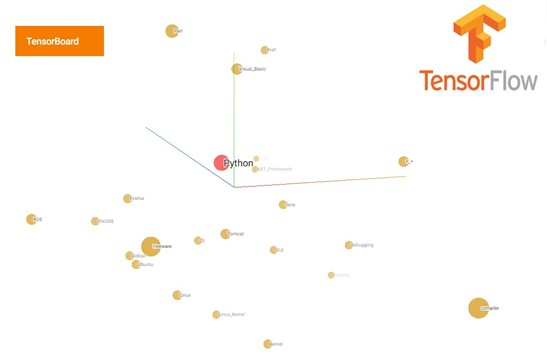Thankfully, Google makes freely available their Word2Vec model that was trained on close to 100 billion words from Google News. We will be visualizing this trained model with Tensorflow's Embedding Projector.

Download the zipped model from here. It is a 1.6GB compressed file.

Then, extract it to a location of your choice. The model is a 3.5GB bin file called: GoogleNews-vectors-negative300.bin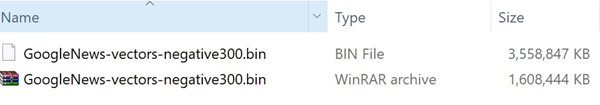Load Word2Vec with Gensim

Gensim is an open source python package for space and topic modeling. Amongst its functionality is a Word2Vec implementation that you can use to train custom Word2Vec models. We will be first loading Google’s trained Word2Vec model with Gensim.

If you don’t have Gensim installed just run the following pip command:

pip install --upgrade gensim

To load Google’s trained model run the following code we first import the necessary packages, in this case Gensim. To import the model, we will need the KeyedVectors module that implements word vectors and their similarity look-ups. Also, specify the base Folder Path variable of where your model is stored. Lastly, run the load_word2vec_format command providing the model path.

import gensim
from gensim.models import Word2Vec,KeyedVectors

#base Folder Path
FOLDER_PATH = "C:/GGL_W2V"

Let’s see how many words this model has by running the following command.

print("Vocabulary Size: {0}".format(len(model.vocab)))

We have a 3 Million word Word2Vec model at our disposal. Thanks Google! So many ideas come to mind to put this model to use…. But, let’s keep focus on visualizing this monster model in the embedding projector.

To view the first 5 words that are in the model run the following python code.

for i,w in enumerate(model.vocab):
print(w)
if i>4:
break

#prints </s>, in, for, that, is, on

Lastly, let's take a look at one embedding and its shape. Will use the word “for”.

model["for"].shape
#(300,)

Google’s Word2Vec model has a 300 feature word embedding which we will be visualizing next.

One last step before we continue, we will create a numpy array of shape (VocabularySize, Embedding_Features) that will store Google’s word embeddings. We will populate this array in the next section as we generate our metadata which are the labels we will be plotting along each point with tensorboard embedding projector.

import numpy as np

#Important Parameters
VOCAB_SIZE = len(model.vocab)
EMBEDDING_DIM = model["is"].shape

w2v = np.zeros((VOCAB_SIZE, EMBEDDING_DIM))

In the Tensorboard Projection Embedding we can provide a metadata file with labels or images that will be plotted along each point in the visualization. The metadata is a .tsv file which we will be creating in the following code.

Our code loops through each word in the model, stores the embedding in our w2v array and adds a line to the .tsv file with the label. Once completed, you can open the .tsv to see all the labels. These align with the row of our w2v array.

You’ll notice below, we are saving this in a subfolder of our FOLDER_PATH variable called tensorboard

with open(tsv_file_path,'w+', encoding='utf-8') as file_metadata:
for i,word in enumerate(model.index2word[:VOCAB_SIZE]):
w2v[i] = model[word]

Visualize Word Embeddings with Tensorflow

Let’s now visualize our word embeddings in tensorboard. First, import the required tensorflow packages. The tensorboard embedding projector package can be found in contrib.tensorboard.plugins.

import tensorflow as tf
from tensorflow.contrib.tensorboard.plugins import projector

TENSORBOARD_FILES_PATH = FOLDER_PATH+"/tensorboard"

Because the model is very large, we will be using placeholders to be able and run a tensorflow graph on the model.

Create a placeholder and assign it to the variable X. The placeholder X_init has shape Vocab_Size and Embedding Dimension. Then, we define a global variable initializer which we need to run in order for our variables to actually hold the assign values. A tensorflow session is then started, passing our initializer and using feed_dict we pass our w2v array to be assigned to our X_init placeholder. Lastly, a tensorflow saver is defined and a writer which will output our graph.

#Tensorflow Placeholders
X_init = tf.placeholder(tf.float32, shape=(VOCAB_SIZE, EMBEDDING_DIM), name="embedding")
X = tf.Variable(X_init)

#Initializer
init = tf.global_variables_initializer()

#Start Tensorflow Session
sess = tf.Session()
sess.run(init, feed_dict={X_init: w2v})

#Instance of Saver, save the graph.
saver = tf.train.Saver()
writer = tf.summary.FileWriter(TENSORBOARD_FILES_PATH, sess.graph)

In the next code block we will configure an embedding projector. We then add an embedding variable and provide the path to our metadata .tsv file we previously generated. Using the projector.visualize_embeddings we write the projector’s configuration file which will be read by tensorboard. Lastly we save a checkpoint and close the session

#Configure a Tensorflow Projector
config = projector.ProjectorConfig()

#Write a projector_config
projector.visualize_embeddings(writer,config)

#save a checkpoint
saver.save(sess, TENSORBOARD_FILES_PATH+'/model.ckpt', global_step = VOCAB_SIZE)

#close the session
sess.close()

Run Tensorboard to Visualize the Word Embeddings

To start tensorboard run the below command in your terminal or command prompt providing the path of where the tensorboard files are saved. In our case it is the value of the TENSORBOARD_FILES_PATH variable.

python -m tensorboard.main --logdir=C:/GGL_W2V/tensorboard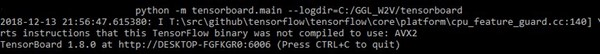Upon successful start, you will see an url that you then browse to access tensorboard. Navigate to the Projector as by clicking on the Projector link as is shown below.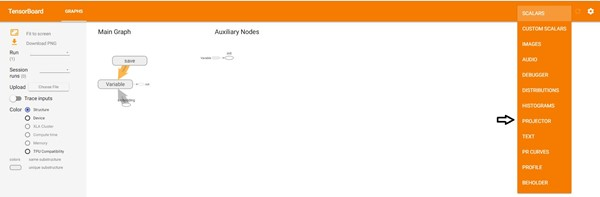You are now ready to visualize your word embeddings using Tensorboard’s Projector plugin.

Below is what a visualization for the word Python looks like filtered on only the most similar words. You can see related words such as Visual_Basic, PHP, Pearl and Java amongst others show up.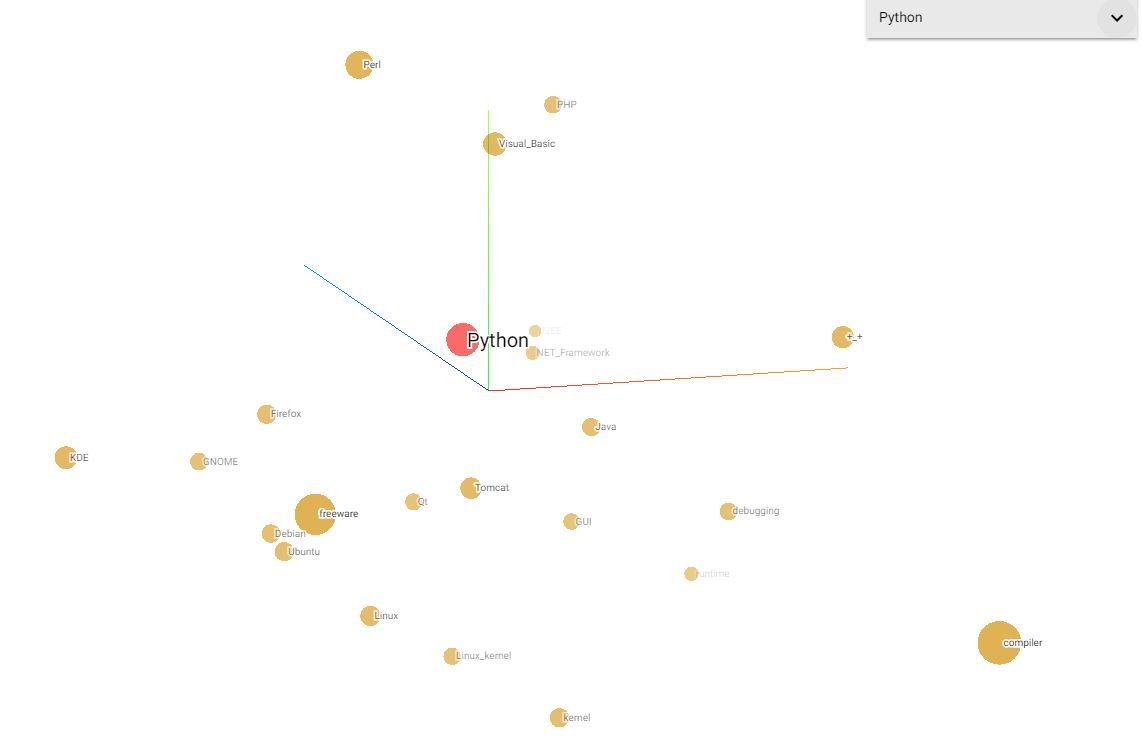Conclusion

With tensorboard we can not only visualize complex neural network graphs but also our Word Embeddings. In this tutorial you learned how to visualize an existing word2vec model. We used google’s pretrained model, loaded it with Gensim and then used Tensorflow to visualize it with the embedding projector plugin. Now, on to play with the visualizations! Stay tuned for more.